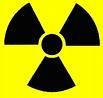# Science Quiz / 'P' & 'Q' Units of Measurement

Random Science or Math Quiz

## Can you name the units of measurement that begin with 'p' and 'q'?

#### byCryptus  Plays Quiz not verified by Sporcle

Score
0/28
Timer
08:00
ClueUnit
A traditional unit of distance, defined as the distance between two successive falls of the same foot of someone walking i.e. 2 steps.
A unit of quantity equal to 2.
A traditional unit of distance based on the width of a person's hand, equal to 4 digits.
An astronomical unit of distance, based on the apparent movement of stars due to the movement of the Earth.
The SI unit of pressure.
A unit used to measure servings of butter.
A unit of volume, used mostly for dry goods such as grains; it is equal to 2 gallons.
A unit of weight in the troy system, equal to 24 grains, or 1/20 of a troy ounce.
A unit of length, equal to 16.5 feet; it is an alternate name for the rod.
A logarithmic measure used to state the acidity or alkalinity of a solution.
The CGS unit of illuminence, equal to one lumen per square centimeter.
An irrational number, used in a unit sense when talking of radians.
A unit of typographical length, equal to 12 points.
A unit of volume for champagne, equal to 1/4 of a bottle.
An informal unit used in cooking, historically defined as an amount that can be taken between the thumb and forefinger.
An imperial unit of volume, equal to 568 millilitres (UK), 473 milliliters (US).
A 'unit' of picture resolution.
A unit representing the point at which gravity becomes quantised; the shortest distance that is meaningful in our current understanding of physics.
A typographical unit of length; originally defined as the smallest element of type that could be handled when printing was done from hand-set metal type.
An imperial unit of weight, equal to 16 ounces.
A unit of proportion used in measuring the strength of alcoholic liquors.
A unit of angle measure equal to 1/4 of a circle.
A unit of relative energy in physics, defined as a discrete packet of energy.
A unit of volume equal to 1/4 of a gallon.
A unit of time used in business, equal to 3 months.
A unit of quantity equal to 4.
A unit of relative time in music, equal to 1/8 of a whole note.
A unit of quantity equal to 5.

## From the Vault See Another

Colored Star Selection III

by goc3

Now would be an appropriate time to shoot for the stars.

### Extras

Created Jan 20, 2016